# Chapter 6 Regression

## 6.1 Correlation

An example from Hays (1974, pp. 633-635):

“The teacher collected data for a class of 91 students, obtaining for each a score X, based on the number of courses in high-school mathematics, and a score Y, the actual score on the final examination for the course.”

Table 6.1: Data for Correlation
X Y
2.0 22
2.0 17
2.0 16
2.0 14
2.0 10
2.0 9
2.0 7
2.0 5
2.0 3
2.0 2
2.5 26
2.5 23
2.5 18
2.5 18
2.5 16
2.5 13
2.5 12
2.5 10
2.5 10
2.5 7
2.5 6
3.0 29
3.0 26
3.0 26
3.0 24
3.0 24
3.0 23
3.0 22
3.0 16
3.0 9
3.0 8
3.5 34
3.5 26
3.5 25
3.5 23
3.5 23
3.5 22
3.5 22
3.5 19
3.5 18
3.5 17
3.5 17
3.5 17
3.5 12
3.5 8
4.0 36
4.0 35
4.0 30
4.0 27
4.0 25
4.0 25
4.0 24
4.0 21
4.0 20
4.0 19
4.0 19
4.0 18
4.0 18
4.0 12
4.0 3
4.5 28
4.5 27
4.5 16
5.0 41
5.0 32
5.0 27
5.0 19
5.5 32
5.5 25
5.5 25
6.0 46
6.0 38
6.0 34
6.0 33
6.0 27
6.0 20
6.5 44
6.5 37
6.5 32
6.5 28
7.0 52
7.0 46
7.0 37
7.5 42
7.5 41
7.5 38
7.5 35
8.0 53
8.0 48
8.0 40
8.0 40

### 6.1.1 Results Overview

Table 6.2: Result Overview Correlation
By Hand JASP SPSS SAS Minitab R
Pearson 0.81 0.8064 0.806 0.8064 0.806 0.8064
Spearman NA 0.7730 0.773 0.7730 0.773 0.7730
Kendall NA 0.6121 0.612 0.6120 NA 0.6120

### 6.1.2 By Hand

Calculations by hand can be found in Hays, 1974, pp. 633-635.

Result: r = 0.81

Note: Hays calculated only the Pearson correlation coefficient.

### 6.1.3 JASP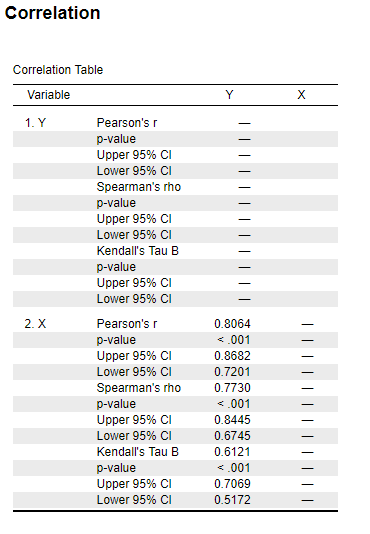Figure 6.1: JASP Output for Correlation

### 6.1.4 SPSS

DATASET ACTIVATE DataSet1.
CORRELATIONS
/VARIABLES=Y X
/PRINT=TWOTAIL NOSIG
/MISSING=PAIRWISE.
NONPAR CORR
/VARIABLES=Y X
/PRINT=BOTH TWOTAIL NOSIG
/MISSING=PAIRWISE.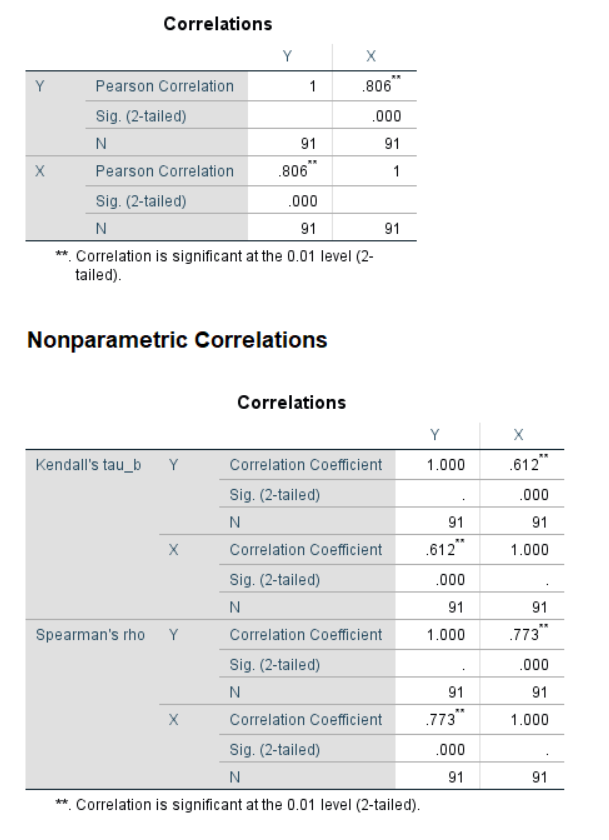Figure 6.2: SPSS Output for Correlation

### 6.1.5 SAS

PROC CORR DATA=Correlation pearson spearman kendall;
VAR X;
WITH Y;
RUN;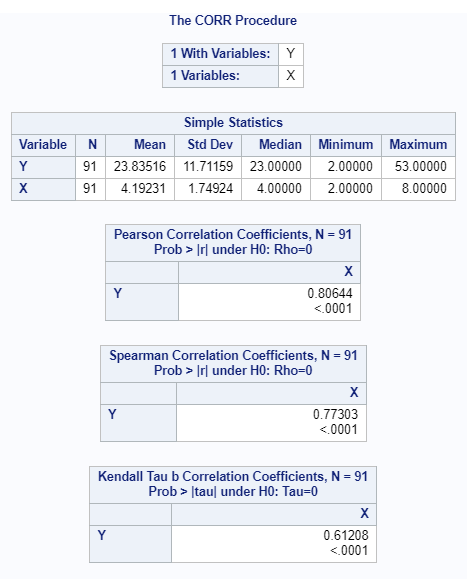Figure 6.3: SAS Output for Correlation

### 6.1.6 Minitab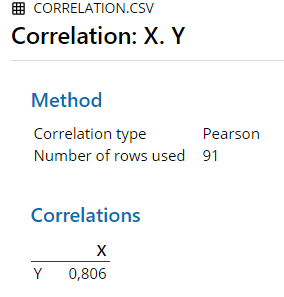Figure 6.4: Minitab Output for Pearson Correlation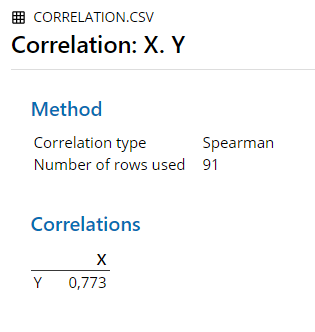Figure 6.5: Minitab Output for Spearman Correlation

### 6.1.7 R

cor.test(corr.data$X, corr.data$Y, method ="pearson")
##
## 	Pearson's product-moment correlation
##
## data:  corr.data$X and corr.data$Y
## t = 12.866, df = 89, p-value < 2.2e-16
## alternative hypothesis: true correlation is not equal to 0
## 95 percent confidence interval:
##  0.7200843 0.8681910
## sample estimates:
##       cor
## 0.8064365
cor.test(corr.data$X, corr.data$Y, method ="spearman")
## Warning in cor.test.default(corr.data$X, corr.data$Y, method = "spearman"):
## Cannot compute exact p-value with ties
##
## 	Spearman's rank correlation rho
##
## data:  corr.data$X and corr.data$Y
## S = 28502, p-value < 2.2e-16
## alternative hypothesis: true rho is not equal to 0
## sample estimates:
##       rho
## 0.7730333
cor.test(corr.data$X, corr.data$Y, method ="kendall")
##
## 	Kendall's rank correlation tau
##
## data:  corr.data$X and corr.data$Y
## z = 8.1519, p-value = 3.582e-16
## alternative hypothesis: true tau is not equal to 0
## sample estimates:
##       tau
## 0.6120845

### 6.1.8 Remarks

All differences in results between the software and hand calculation are due to rounding.

### 6.1.9 References

Hays, W. L. (1974). Statistics for the social sciences (2nd Ed.). New York, US: Holt, Rinehart and Winston, Inc.

## 6.2 Linear Regression

An example from Hays (1974, pp. 633-635):

“The teacher collected data for a class of 91 students, obtaining for each a score X, based on the number of courses in high-school mathematics, and a score Y, the actual score on the final examination for the course.”

Table 6.3: Data for Regression
X Y
2.0 22
2.0 17
2.0 16
2.0 14
2.0 10
2.0 9
2.0 7
2.0 5
2.0 3
2.0 2
2.5 26
2.5 23
2.5 18
2.5 18
2.5 16
2.5 13
2.5 12
2.5 10
2.5 10
2.5 7
2.5 6
3.0 29
3.0 26
3.0 26
3.0 24
3.0 24
3.0 23
3.0 22
3.0 16
3.0 9
3.0 8
3.5 34
3.5 26
3.5 25
3.5 23
3.5 23
3.5 22
3.5 22
3.5 19
3.5 18
3.5 17
3.5 17
3.5 17
3.5 12
3.5 8
4.0 36
4.0 35
4.0 30
4.0 27
4.0 25
4.0 25
4.0 24
4.0 21
4.0 20
4.0 19
4.0 19
4.0 18
4.0 18
4.0 12
4.0 3
4.5 28
4.5 27
4.5 16
5.0 41
5.0 32
5.0 27
5.0 19
5.5 32
5.5 25
5.5 25
6.0 46
6.0 38
6.0 34
6.0 33
6.0 27
6.0 20
6.5 44
6.5 37
6.5 32
6.5 28
7.0 52
7.0 46
7.0 37
7.5 42
7.5 41
7.5 38
7.5 35
8.0 53
8.0 48
8.0 40
8.0 40

### 6.2.1 Results Overview

Table 6.4: Result Overview Independent Factorial ANOVA
By Hand JASP SPSS SAS Minitab R
Constant 23.84 23.8352 23.835 23.8352 23.835 23.8350
Regression Coefficient 5.42 5.3993 5.399 5.3993 5.399 5.3990
$$\overline{x}$$ 4.19 4.1923 4.192 4.1923 4.192 4.1923

### 6.2.2 By Hand

Calculations by hand can be found in Hays, 1974, pp. 633-635.

Results:

y’ = (5.42)(x - 4.19) + 23.84

Note: Hays mean-centered the equation by the mean of $$\overline{x}$$ = 4.19.

### 6.2.3 JASP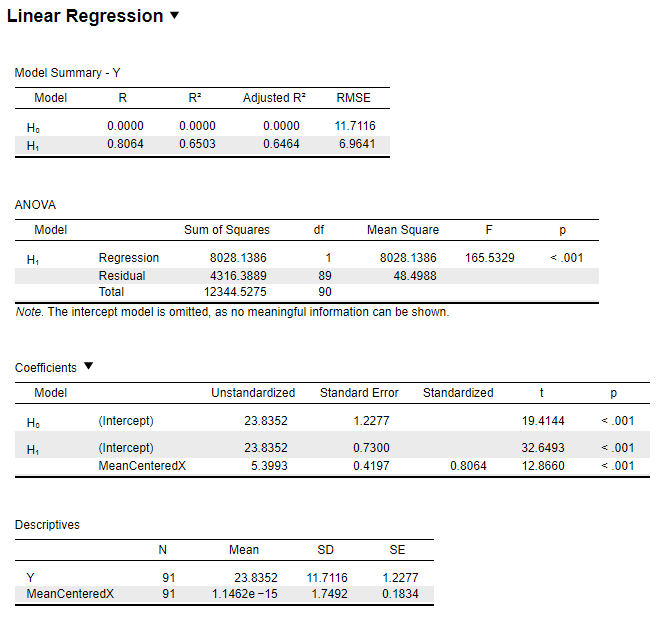Figure 6.6: JASP Output for Regression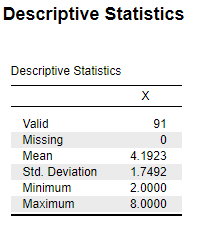Figure 6.7: JASP Output for Descriptives

Mean-centered regression equation:

y’ = (5.3993)(x - 4.1923) + 23.8352

### 6.2.4 SPSS

DESCRIPTIVES VARIABLES=X
/STATISTICS=MEAN.

REGRESSION
/DESCRIPTIVES MEAN STDDEV CORR SIG N
/MISSING LISTWISE
/STATISTICS COEFF OUTS R ANOVA
/CRITERIA=PIN(.05) POUT(.10)
/NOORIGIN
/DEPENDENT Y
/METHOD=ENTER MeanCenteredX.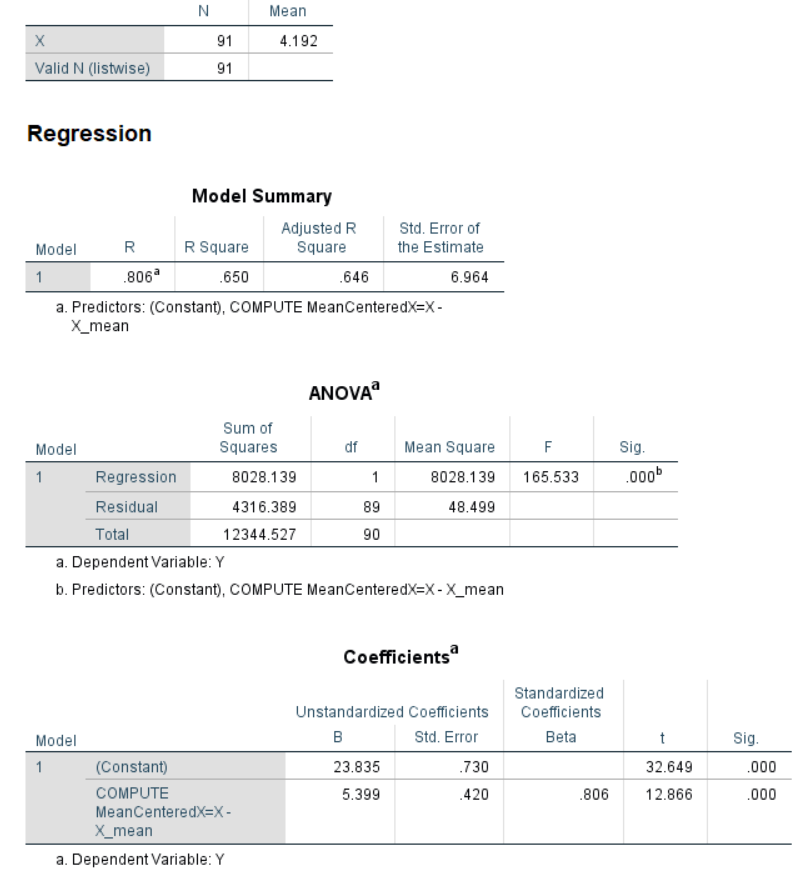Figure 6.8: SPSS Output for Regression

Mean-centered regression equation:

y’ = (5.399)(x - 4.192) + 23.835

### 6.2.5 SAS

proc Reg data=Regression;
title "Linear regression";
model Y = MeanCenteredX;
run;

PROC MEANS DATA=Regression;
VAR X Y;
RUN;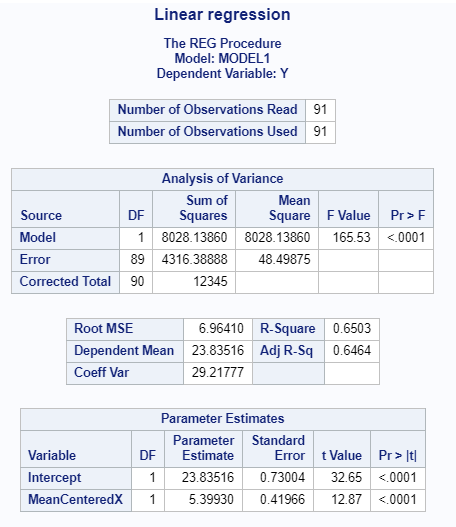Figure 6.9: SAS Output for Regression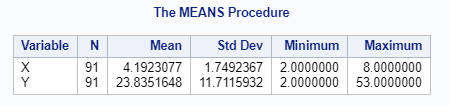Figure 6.10: SAS Output for Means

Mean-centered regression equation:

y’ = (5.3993)(x - 4.1923077) + 23.83516

### 6.2.6 Minitab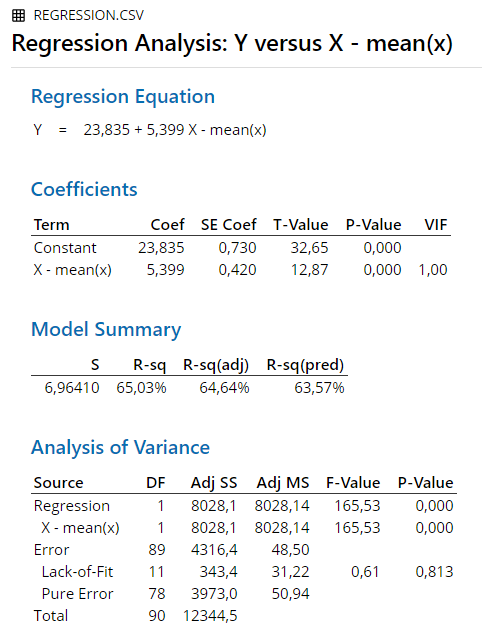Figure 6.11: Minitab Output for Regression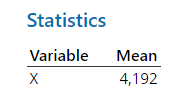Figure 6.12: Minitab Output for Means

Mean-centered regression equation:

y’ = (5.399)(x - 4.192) + 23.835

### 6.2.7 R

regress.data2 <- read.csv("Datasets/Regression.csv", sep=",")
lm(formula = Y ~ MeanCenteredX, data = regress.data2)
##
## Call:
## lm(formula = Y ~ MeanCenteredX, data = regress.data2)
##
## Coefficients:
##   (Intercept)  MeanCenteredX
##        23.835          5.399
mean(regress.data2\$X)
##  4.192308

Mean-centered regression equation:

y’ = (5.399)(x - 4.192308) + 23.835

### 6.2.8 Remarks

All differences in results between the software and hand calculation are due to rounding.

### 6.2.9 References

Hays, W. L. (1974). Statistics for the social sciences (2nd Ed.). New York, US: Holt, Rinehart and Winston, Inc.

## 6.3 Logistic Regression

An example:

The Titanic-dataset contains original data of all passengers of the Titanic. It contains their name, their passenger class (1st - 3rd), their age, their sex, and whether or not they survived the sinking of the ship. The logistic regression model is computed to allow predictions on a passengers survival status, based on their age, sex, and passenger class.

### 6.3.1 Results Overview

Table 6.5: Result Overview Coefficents Logistic Regression
JASP SPSS SAS Minitab R
Constant 3.7597 3.760 3.7596 3.7600 3.7597
Age -0.0392 -0.039 -0.0392 -0.0392 -0.0392
2nd Class -1.2920 -1.292 -1.2920 -1.2920 -1.2920
3rd Class -2.5214 -2.521 -2.5214 -2.5210 -2.5214
Sex(Male) -2.6314 -2.631 -2.6313 -2.6310 -2.6314
Table 6.6: Result Overview Odds-ratio Logistic Regression
JASP SPSS SAS Minitab R
Constant 42.9339 42.934 NA NA 42.9339
Age 0.9616 0.962 0.962 0.9616 0.9616
2nd Class 0.2747 0.275 0.275 0.2747 0.2747
3rd Class 0.0803 0.080 0.080 0.0803 0.0803
Sex(Male) 0.0720 0.072 0.072 0.0720 0.0720

Note: The reference case to which the odds ratios are refering is a female passenger in the first class with age 0.

### 6.3.2 JASP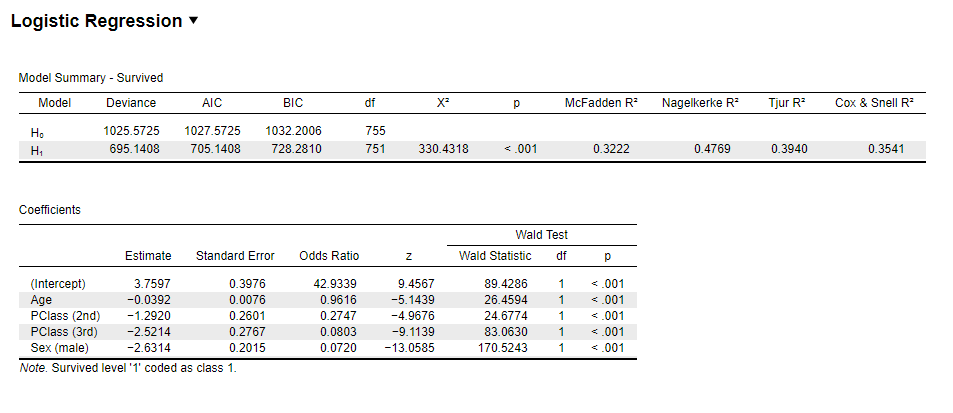Figure 6.13: JASP Output for Logistic Regression

### 6.3.3 SPSS

DATASET ACTIVATE DataSet1.
LOGISTIC REGRESSION VARIABLES Survived
/METHOD=ENTER PClass Age Sex
/CONTRAST (PClass)=Indicator(1)
/CONTRAST (Sex)=Indicator(1)
/CRITERIA=PIN(.05) POUT(.10) ITERATE(20) CUT(.5).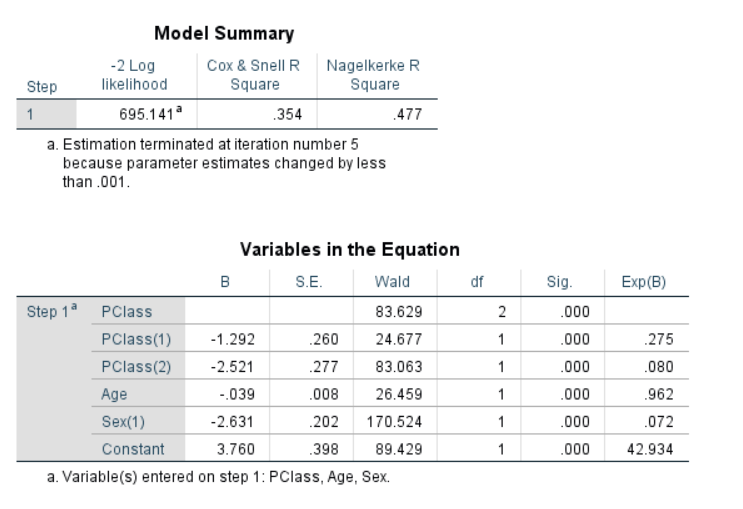Figure 6.14: SPSS Output for Logistic Regression

### 6.3.4 SAS

proc logistic data=work.LogReg DESC;
class PClass Sex / param=reference ref=first;
model Survived = Age Sex PClass;
run;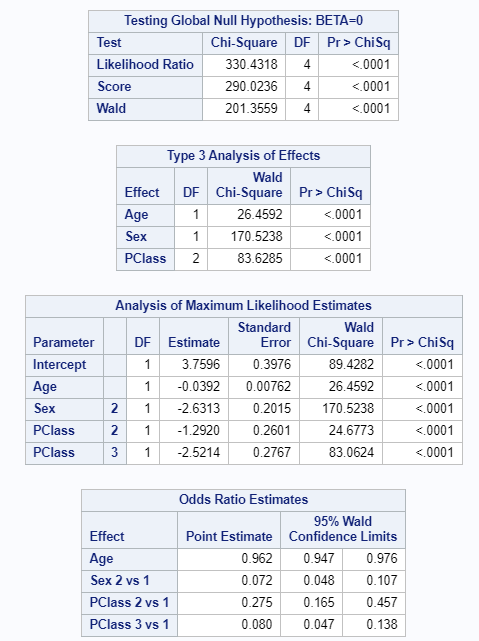Figure 6.15: SAS Output for Logistic Regression

### 6.3.5 Minitab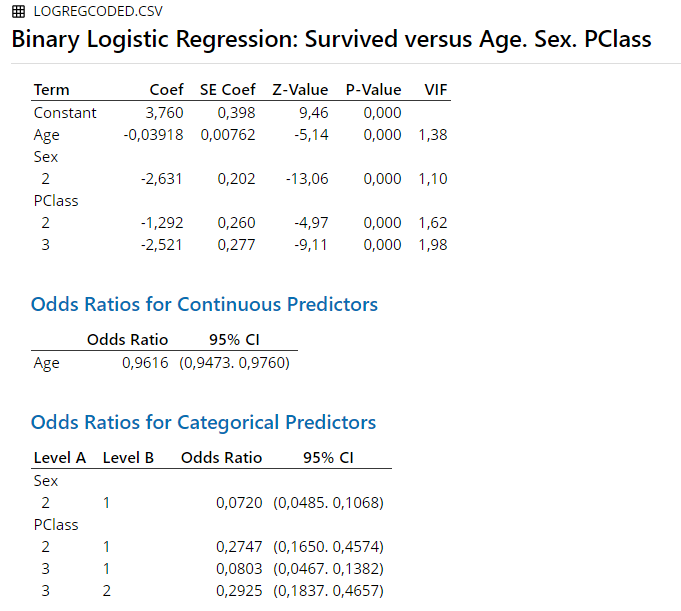Figure 6.16: Minitab Output for Logistic Regression

### 6.3.6 R

LogRegExample <- glm(factor(Survived) ~Age + factor(PClass) + factor(Sex), data=LogReg.data2, family=binomial(link="logit"))
summary(LogRegExample)
##
## Call:
## glm(formula = factor(Survived) ~ Age + factor(PClass) + factor(Sex),
##     family = binomial(link = "logit"), data = LogReg.data2)
##
## Deviance Residuals:
##     Min       1Q   Median       3Q      Max
## -2.7226  -0.7065  -0.3917   0.6495   2.5289
##
## Coefficients:
##                  Estimate Std. Error z value Pr(>|z|)
## (Intercept)      1.172856   0.253988   4.618 3.88e-06 ***
## Age             -0.039177   0.007616  -5.144 2.69e-07 ***
## factor(PClass)1  1.271127   0.160563   7.917 2.44e-15 ***
## factor(PClass)2 -0.020835   0.138004  -0.151     0.88
## factor(Sex)1     1.315678   0.100753  13.058  < 2e-16 ***
## ---
## Signif. codes:  0 '***' 0.001 '**' 0.01 '*' 0.05 '.' 0.1 ' ' 1
##
## (Dispersion parameter for binomial family taken to be 1)
##
##     Null deviance: 1025.57  on 755  degrees of freedom
## Residual deviance:  695.14  on 751  degrees of freedom
##   (557 observations deleted due to missingness)
## AIC: 705.14
##
## Number of Fisher Scoring iterations: 5
exp(coef(LogRegExample))
##     (Intercept)             Age factor(PClass)1 factor(PClass)2    factor(Sex)1
##       3.2312094       0.9615807       3.5648686       0.9793803       3.7272788

### 6.3.7 Remarks

All differences in results between the software are due to rounding.